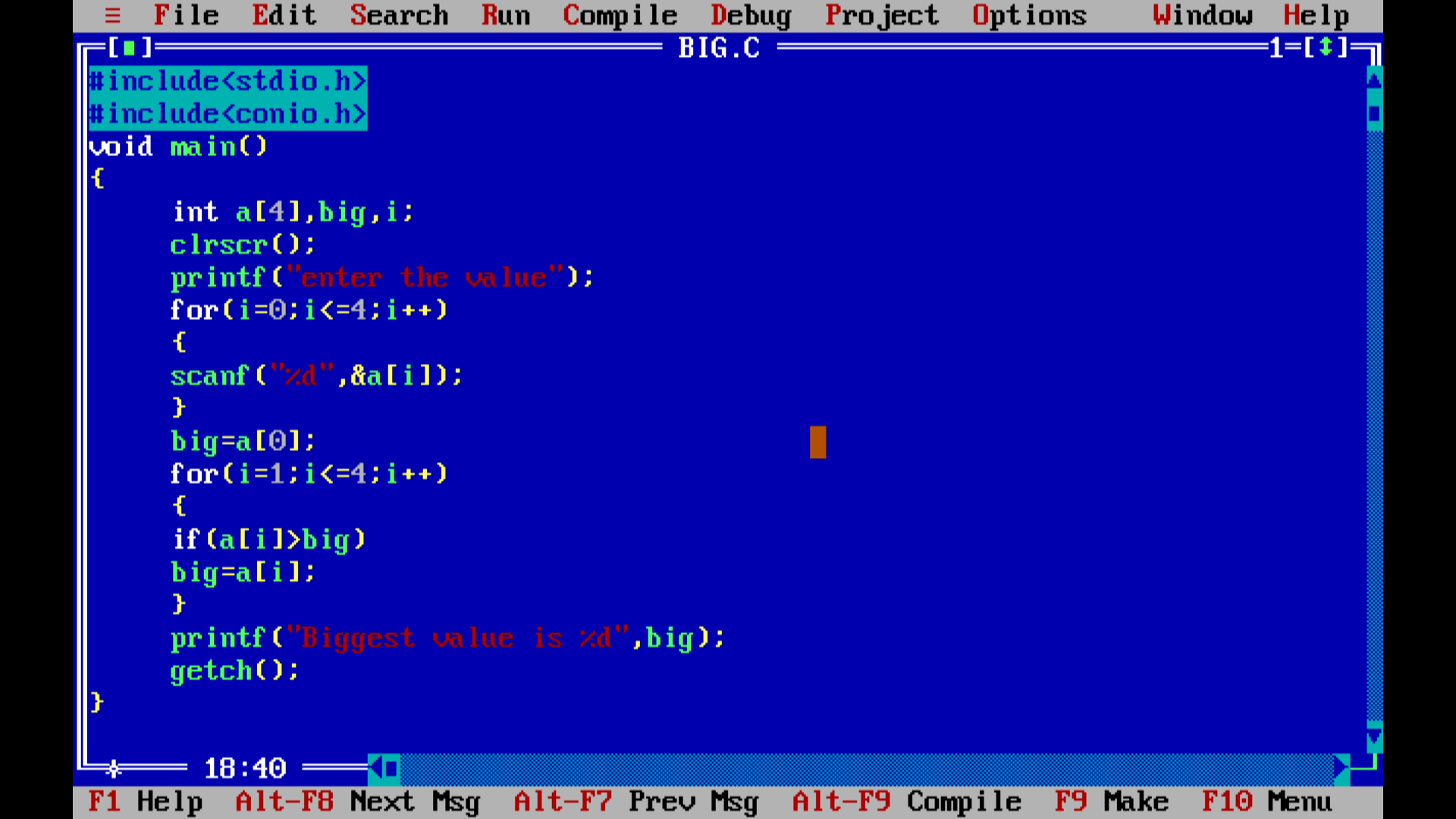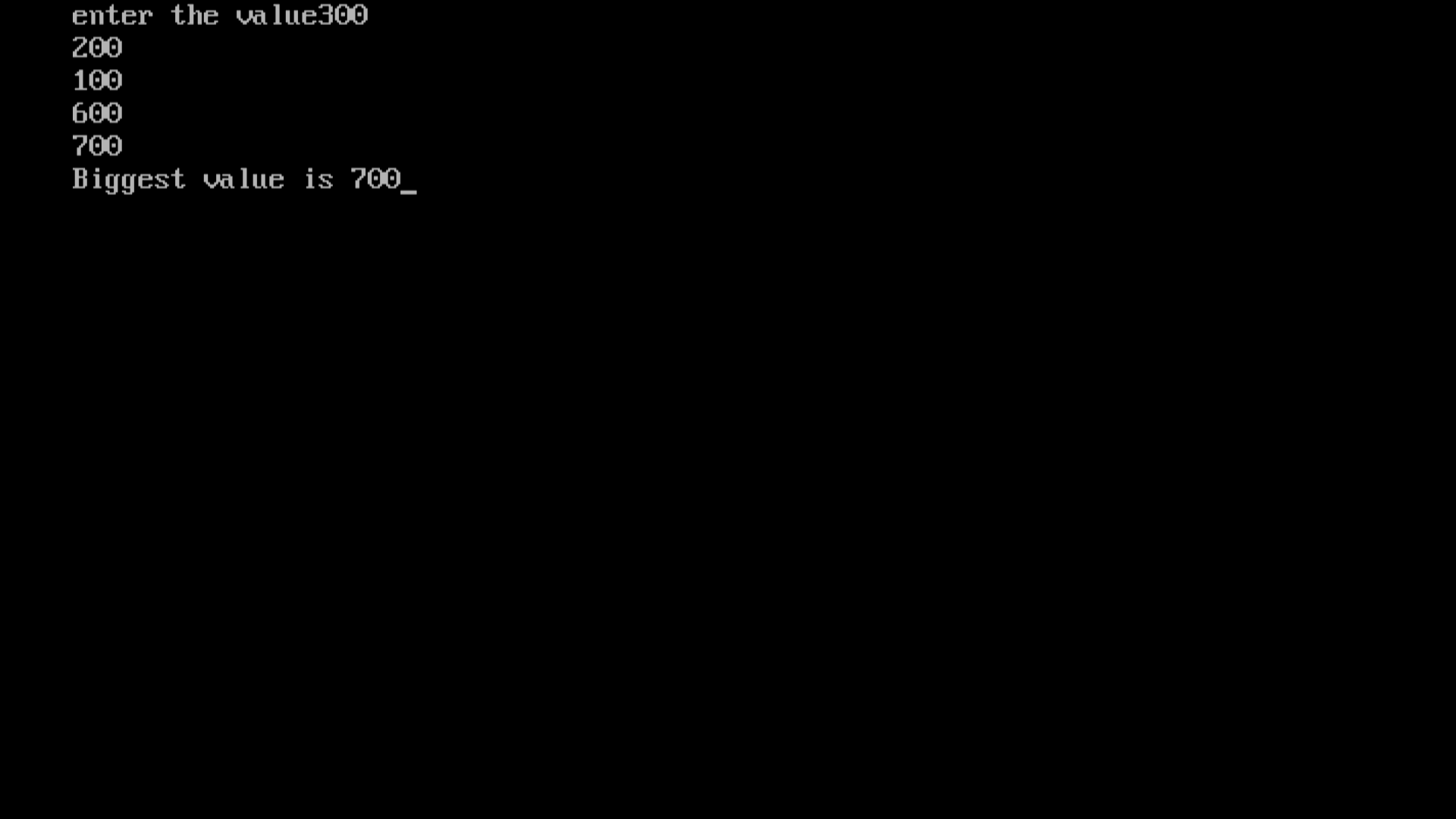Article

# Assigment-folder(To find the biggest number in a 1D array in C,)

To find the biggest number in a 1D array in C, you can iterate through the array and keep track of the maximum value encountered.``````
#include<stdio.h>
#include<conio.h>
void main()
{
int a,big,i;
clrscr();
printf("enter the value");
for(i=0;i<=4;i++)
{
scanf("%d",&a[i]);
}
big=a;
for(i=1;i<=4;i++)
{
if(a[i]>big)
big=a[i];
}
printf("Biggest value is %d",big);
getch();
}
``````
````In this example, we start by initializing the big variable to the first element of the array. Then, we loop through the array, comparing each element to the current maximum (big). If we find an element greater than big, we update big to that element. Finally, after the loop, big will contain the largest number in the array.Make sure to replace the a[i] array with your own array if you want to find the largest number in a different set of numbers.`
```